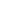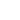AI

# 构建简单数据管道，为什么tf.data要比feed_dict更好？`tf.data`管道没有这个问题，它能提前抓取下个batch的数据，降低总体闲置时间。在这个基础上，如果我们采用并行数据导入，或者事先进行数据预处理，整个过程就更快了。#### 在5分钟内实现小型图像管道

``````[
[Tensor(image), Tensor(label)],
[Tensor(image), Tensor(label)],
...
]
``````

• Dataset(list of image files) → Dataset(actual images)

• Dataset(6400 images) → Dataset(64 batches with 100 images each)

• Dataset(list of audio files) → Dataset(shuffled list of audio files)``````# define list of files
files = ['a.png', 'b.png', 'c.png', 'd.png']

# create a dataset from filenames
dataset = tf.data.Dataset.from_tensor_slices(files)
``````

``````# Source

# Don't use tf.image.decode_image, or the output shape will be undefined
image = tf.image.decode_jpeg(image_string, channels=3)

# This will convert to float values in [0, 1]
image = tf.image.convert_image_dtype(image, tf.float32)

image = tf.image.resize_images(image, [image_size, image_size])
return image

# Apply the function load_image to each filename in the dataset
``````

``````# Create batches of 64 images each
dataset = dataset.batch(64)
``````

`dataset = dataset.prefetch(buffer_size=1)`

``````iterator = dataset.make_initializable_iterator()
``````

``````batch_of_images = iterator.get_next()
``````

``````with tf.Session() as session:

for i in range(epochs):
session.run(iterator.initializer)

try:
# Go through the entire dataset
while True:
image_batch = session.run(batch_of_images)

except tf.errors.OutOfRangeError:
print('End of Epoch.')
``````

nvidia-smi这个命令可以帮我们监控GPU利用率，找到数据管道中的瓶颈。正常情况下，GPU的平均利用率应该高于70%-80%。

#### 更完整的数据管道Shuffle

`Dataset`里，`tf.data.Dataset.shuffle()`是一个比较常用的方法，它可以用来打乱数据集中的数据顺序。它的参数`buffer_size`指定的是一次打乱的元素数量，一般情况下，我们建议把这个参数值设大一点，最好一次性就能把整个数据集洗牌，因为如果参数过小，它可能会造成意料之外的偏差。

``````dataset = tf.data.Dataset.from_tensor_slices(files)
dataset = dataset.shuffle(len(files))
``````

``````# Source
def train_preprocess(image):
image = tf.image.random_flip_left_right(image)

image = tf.image.random_brightness(image, max_delta=32.0 / 255.0)
image = tf.image.random_saturation(image, lower=0.5, upper=1.5)
# Make sure the image is still in [0, 1]
image = tf.clip_by_value(image, 0.0, 1.0)

return image
``````

``````# files is a python list of image filenames
# labels is a numpy array with label data for each image
dataset = tf.data.Dataset.from_tensor_slices((files, labels))
``````

``````def load_image(path, label):

return image, label

``````

### 评论游客2017-09-21

2017-12-01

2017-08-03

2017-08-18

### 迁移学习全面指南：概念、应用、优势、挑战发布需求联系客服